# Statistics review 9: One-way analysis of variance

## Abstract

This review introduces one-way analysis of variance, which is a method of testing differences between more than two groups or treatments. Multiple comparison procedures and orthogonal contrasts are described as methods for identifying specific differences between pairs of treatments.

## Introduction

Analysis of variance (often referred to as ANOVA) is a technique for analyzing the way in which the mean of a variable is affected by different types and combinations of factors. One-way analysis of variance is the simplest form. It is an extension of the independent samples t-test (see statistics review 5 ) and can be used to compare any number of groups or treatments. This method could be used, for example, in the analysis of the effect of three different diets on total serum cholesterol or in the investigation into the extent to which severity of illness is related to the occurrence of infection.

Analysis of variance gives a single overall test of whether there are differences between groups or treatments. Why is it not appropriate to use independent sample t-tests to test all possible pairs of treatments and to identify differences between treatments? To answer this it is necessary to look more closely at the meaning of a P value.

When interpreting a P value, it can be concluded that there is a significant difference between groups if the P value is small enough, and less than 0.05 (5%) is a commonly used cutoff value. In this case 5% is the significance level, or the probability of a type I error. This is the chance of incorrectly rejecting the null hypothesis (i.e. incorrectly concluding that an observed difference did not occur just by chance ), or more simply the chance of wrongly concluding that there is a difference between two groups when in reality there no such difference.

If multiple t-tests are carried out, then the type I error rate will increase with the number of comparisons made. For example, in a study involving four treatments, there are six possible pairwise comparisons. (The number of pairwise comparisons is given by 4C2 and is equal to 4!/ [2!2!], where 4! = 4 × 3 × 2 × 1.) If the chance of a type I error in one such comparison is 0.05, then the chance of not committing a type I error is 1 - 0.05 = 0.95. If the six comparisons can be assumed to be independent (can we make a comment or reference about when this assumption cannot be made?), then the chance of not committing a type I error in any one of them is 0.956 = 0.74. Hence, the chance of committing a type I error in at least one of the comparisons is 1 - 0.74 = 0.26, which is the overall type I error rate for the analysis. Therefore, there is a 26% overall type I error rate, even though for each individual test the type I error rate is 5%. Analysis of variance is used to avoid this problem.

## One-way analysis of variance

In an independent samples t-test, the test statistic is computed by dividing the difference between the sample means by the standard error of the difference. The standard error of the difference is an estimate of the variability within each group (assumed to be the same). In other words, the difference (or variability) between the samples is compared with the variability within the samples.

In one-way analysis of variance, the same principle is used, with variances rather than standard deviations being used to measure variability. The variance of a set of n values (x1, x2 ... xn) is given by the following (i.e. sum of squares divided by the degrees of freedom):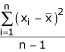Where the sum of squares =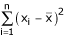and the degrees of freedom = n - 1

Analysis of variance would almost always be carried out using a statistical package, but an example using the simple data set shown in Table 1 will be used to illustrate the principles involved.

The grand mean of the total set of observations is the sum of all observations divided by the total number of observations. For the data given in Table 1, the grand mean is 16. For a particular observation x, the difference between x and the grand mean can be split into two parts as follows:

x - grand mean = (treatment mean - grand mean) + (x - treatment mean)

Total deviation = deviation explained by treatment + unexplained deviation (residual)

This is analogous to the regression situation (see statistics review 7 ) with the treatment mean forming the fitted value. This is shown in Table 2.

The total sum of squares for the data is similarly partitioned into a 'between treatments' sum of squares and a 'within treatments' sum of squares. The within treatments sum of squares is also referred to as the error or residual sum of squares.

The degrees of freedom (df) for these sums of squares are as follows:

Total df = n - 1 (where n is the total number of observations) = 9 - 1 = 8

Between treatments df = number of treatments - 1 = 3 - 1 = 2

Within treatments df = total df - between treatments df = 8 - 2 = 6

This partitioning of the total sum of squares is presented in an analysis of variance table (Table 3). The mean squares (MS), which correspond to variance estimates, are obtained by dividing the sums of squares (SS) by their degrees of freedom.

The test statistic F is equal to the 'between treatments' mean square divided by the error mean square. The P value may be obtained by comparison of the test statistic with the F distribution with 2 and 6 degrees of freedom (where 2 is the number of degrees of freedom for the numerator and 6 for the denominator). In this case it was obtained from a statistical package. The P value of 0.0039 indicates that at least two of the treatments are different.

As a published example we shall use the results of an observational study into the prevalence of infection among intensive care unit (ICU) patients. One aspect of the study was to investigate the extent to which severity of illness was related to the occurrence of infection. Patients were categorized according to the presence of infection. The categories used were no infection, infection on admission, ICU-acquired infection, and both infection on admission and ICU-acquired infection. (These are referred to as infection states 1–4.) To assess the severity of illness, the Simplified Acute Physiology Score (SAPS) II system was used . Findings in 400 patients (100 in each category) were analyzed. (It is not necessary to have equal sample sizes.) Table 4 shows some of the scores together with the sample means and standard deviations for each category of infection. The whole data set is illustrated in Fig. 1 using box plots.

The analysis of variance output using a statistical package is shown in Table 5.

## Multiple comparison procedures

When a significant effect has been found using analysis of variance, we still do not know which means differ significantly. It is therefore necessary to conduct post hoc comparisons between pairs of treatments. As explained above, when repeated t-tests are used, the overall type I error rate increases with the number of pairwise comparisons. One method of keeping the overall type I error rate to 0.05 would be to use a much lower pairwise type I error rate. To calculate the pairwise type I error rate α needed to maintain a 0.05 overall type I error rate in our four observational group example, we use 1 - (1 - α)N = 0.05, where N is the number of possible pairwise comparisons. In this example there were four means, giving rise to six possible comparisons. Rearranging this gives α = 1 - (0.95)1/6 = 0.0085. A method of approximating this calculated value is attributed to Bonferoni. In this method the overall type I error rate is divided by the number of comparisons made, to give a type I error rate for the pairwise comparison. In our four treatment example, this would be 0.05/6 = 0.0083, indicating that a difference would only be considered significant if the P value were below 0.0083. The Bonferoni method is often regarded as too conservative (i.e. it fails to detect real differences).

There are a number of specialist multiple comparison tests that maintain a low overall type I error. Tukey's test and Duncan's multiple-range test are two of the procedures that can be used and are found in most statistical packages.

## Duncan's multiple-range test

We use the data given in Table 4 to illustrate Duncan's multiple-range test. This procedure is based on the comparison of the range of a subset of the sample means with a calculated least significant range. This least significant range increases with the number of sample means in the subset. If the range of the subset exceeds the least significant range, then the population means can be considered significantly different. It is a sequential test and so the subset with the largest range is compared first, followed by smaller subsets. Once a range is found not to be significant, no further subsets of this group are tested.

The least significant range, Rp, for subsets of p sample means is given by: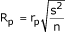Where rp is called the least significant studentized range and depends upon the error degrees of freedom and the numbers of means in the subset. Tables of these values can be found in many statistics books ; s2 is the error mean square from the analysis of variance table, and n is the sample size for each treatment. For the data in Table 4, s2 = 208.9, n = 100 (if the sample sizes are not equal, then n is replaced with the harmonic mean of the sample sizes ) and the error degrees of freedom = 396. So, from the table of studentized ranges , r2 = 2.77, r3 = 2.92 and r4 = 3.02. The least significant range (Rp) for subsets of 2, 3 and 4 means are therefore calculated as R2 = 4.00, R3 = 4.22 and R4 = 4.37.

To conduct pairwise comparisons, the sample means must be ordered by size: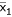= 35.2,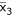= 39.4,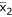= 39.5 and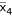= 40.9

The subset with the largest range includes all four infections, and this will compare infection 4 with infection 1. The range of that subset is the difference between the sample means-= 5.7. This is greater than the least significant range R4 = 4.37, and therefore it can be concluded that infection state 4 is associated with significantly higher SAPS II scores than infection state 1.

Sequentially, we now need to compare subsets of three groups (i.e. infection state 2 with infection state 1, and infection state 4 with infection state 3):-= 4.3 and-= 1.5. The difference of 4.3 is greater than R3 = 4.22, showing that infection state 2 is associated with a significantly higher SAPS II score than is infection state 1. The difference of 1.5, being less than 4.33, indicates that there is no significant difference between infection states 4 and 3.

As the range of infection states 4 to 3 was not significant, no smaller subsets within that range can be compared. This leaves a single two-group subset to be compared, namely that of infection 3 with infection 1:-= 4.2. This difference is greater than R2 = 4.00, and therefore it can be concluded that there is a significant difference between infection states 3 and 1. In conclusion, it appears that infection state 1 (no infection) is associated with significantly lower SAPS II scores than the other three infection states, which are not significantly different from each other.

Table 6 gives the output from a statistical package showing the results of Duncan's multiple-range test on the data from Table 4.

## Contrasts

In some investigations, specific comparisons between sets of means may be suggested before the data are collected. These are called planned or a priori comparisons. Orthogonal contrasts may be used to partition the treatment sum of squares into separate components according to the number of degrees of freedom. The analysis of variance for the SAPS II data shown in Table 5 gives a between infection state, sum of squares of 1780.2 with three degrees of freedom. Suppose that, in advance of carrying out the study, it was required to compare the SAPS II scores of patients with no infection with the other three infection categories collectively. We denote the true population mean SAPS II scores for the four infection categories by μ1, μ2, μ3 and μ4, with μ1 being the mean for the no infection group. The null hypothesis states that the mean for the no infection group is equal to the average of the other three means. This can be written as follows:

μ1 = (μ2 + μ3 + μ 4)/3 (i.e. 3μ 1 - μ 2 - μ 3 - μ 4 = 0)

The coefficients of μ1, μ2, μ3 and μ4 (3, -1, -1 and -1) are called the contrast coefficients and must be specified in a statistical package in order to conduct the hypothesis test. Each contrast of this type (where differences between means are being tested) has one degree of freedom. For the SAPS II data, two further contrasts, which are orthogonal (i.e. independent), are therefore possible. These could be, for example, a contrast between infection states 3 and 4, and a contrast between infection state 2 and infection states 3 and 4 combined. The coefficients for these three contrasts are given in Table 7.

The calculation of the contrast sum of squares has been conducted using a statistical package and the results are shown in Table 8. The sums of squares for the contrasts add up to the infection sum of squares. Contrast 1 has a P value of 0.006, indicating a significant difference between the no infection group and the other three infection groups collectively. The other two contrasts are not significant.

## Polynomial contrasts

Where the treatment levels have a natural order and are equally spaced, it may be of interest to test for a trend in the treatment means. Again, this can be carried out using appropriate orthogonal contrasts. For example, in an investigation to determine whether the plasma colloid osmotic pressure (COP) of healthy infants was related to age, the plasma COP of 10 infants from each of three age groups, 1–4 months, 5–8 months and 9–12 months, was measured. The data are given in Table 9 and illustrated in Fig. 2.

With three age groups we can test for a linear and a quadratic trend. The orthogonal contrasts for these trends are set up as shown in Table 10. The linear contrast compares the lowest with the highest age group, and the quadratic contrast compares the middle age group with the lowest and highest age groups together.

The analysis of variance with the tests for the trends is given in Table 11. The P value of 0.138 indicates that there is no overall difference between the mean plasma COP levels at each age group. However, the linear contrast with a P value of 0.049 indicates that there is a significant linear trend, suggesting that plasma COP does increase with age in infants. The quadratic contrast is not significant.

## Assumptions and limitations

The underlying assumptions for one-way analysis of variance are that the observations are independent and randomly selected from Normal populations with equal variances. It is not necessary to have equal sample sizes.

The assumptions can be assessed by looking at plots of the residuals. The residuals are the differences between the observed and fitted values, where the fitted values are the treatment means. Commonly, a plot of the residuals against the fitted values and a Normal plot of residuals are produced. If the variances are equal then the residuals should be evenly scattered around zero along the range of fitted values, and if the residuals are Normally distributed then the Normal plot will show a straight line. The same methods of assessing the assumptions are used in regression and are discussed in statistics review 7 .

If the assumptions are not met then it may be possible to transform the data. Alternatively the Kruskal-Wallis nonparametric test could be used. This test will be covered in a future review.

Figs 3 and 4 show the residual plots for the data given in Table 4. The plot of fitted values against residuals suggests that the assumption of equal variance is reasonable. The Normal plot suggests that the distribution of the residuals is approximately Normal.

## Conclusion

One-way analysis of variance is used to test for differences between more than two groups or treatments. Further investigation of the differences can be carried out using multiple comparison procedures or orthogonal contrasts.

Data from studies with more complex designs can also be analyzed using analysis of variance (e.g. see Armitage and coworkers  or Montgomery ).

## Abbreviations

COP:

colloid osmotic pressure

df:

degrees of freedom

ICU:

intensive care unit

SAPS:

Simplified Acute Physiology Score.

## References

1. Whitely E, Ball J: Statistics review 5: Comparison of means. Crit Care 2002, 6: 424-428. 10.1186/cc1548

2. Bland M: An Introduction to Medical Statistics 3 Edition Oxford, UK: Oxford University Press 2001.

3. Bewick V, Cheek L, Ball J: Statistics review 7: Correlation and Regression. Crit Care 2003, 7: 451-459. 10.1186/cc2401

4. Le Gall JR, Lemeshow S, Saulnier F: A new simplified acute physiology score (SAPS II) based on a European/North American multicenter study. JAMA 1993, 270: 2957-2963. 10.1001/jama.270.24.2957

5. Montgomery DC: Design and Analysis of Experiments 4 Edition New York, USA: Wiley 1997.

6. Armitage P, Berry G, Matthews JNS: Statistical Methods in Medical Research 4 Edition Oxford, UK: Blackwell Science 2002.

## Author information

Authors

### Corresponding author

Correspondence to Viv Bewick.

None declared.

## Rights and permissions

Reprints and Permissions